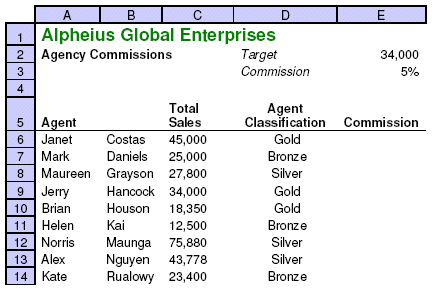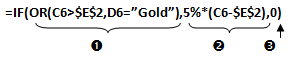# Logical functions

There are several logical functions in Excel. This guide provides details of the 3 most common logical functions:

• IF – used to return one value if the result of a test is true and another value if the result of a test is false.
• AND – used in conjunction with the IF function to allow multiple tests to be performed, then returning one value if ALL the results of the tests are true and another value if the results of ALL the tests are false.
• OR – used in conjunction with the IF function to allow multiple tests to be performed, then returning one value if ANY of the results of the tests are true and another value if the results of ANY of the tests are false.

## IF function

The IF function uses 3 arguments as follows:Operator Action > Greater than < Less than = Equal to <> Not equal to <= Less than or equal to >= Greater than or equal to

1. The test – comparing one value to another using the above operators
2. The value to be displayed where the result of the test is true
3. The value to be displayed where the result of the test is false

### Example – performing a single test

A worksheet lists sales agent names and their annual sales. If the sales agents’ sales have exceeded a specific sales target, then text is to be displayed as “Exceeded”, otherwise text is to be displayed as “Not Exceeded”.

An IF function can be used to display either text result in column D, based on a test to compare each agents sales to the target of \$34,000 in cell E2.The function in cell D5 would be as follows:Note that if text is to be displayed it must have the double quotation marks (“”) around the text in the function.

1. The test comparing the agents’ sales to the target of \$34,000
2. The result to be displayed if the agents’ sales exceed the target
3. The result to be displayed if the agents’ sales is less than the target

### Example – performing more than one test

If you need to perform more than one test when constructing an IF function, you can use either an AND function or an OR function (see above for definitions).

## AND function

In the example below we need to create a function that will calculate the agents’ commission, but only if they have a) exceeded the sales target AND b) have a Gold classification:The formula in cell E3 would be as follows:1. Two tests (separate tests by a comma)
2. The result to be displayed if both the 2 tests are true
3. The result to be displayed if both the tests are not true

This example would only result in Janet Costas getting commission as she was the only agent to have Gold classification and exceeded the target.

## OR function

In the example below we need to create a function that will calculate the agents’ commission if they have EITHER a) exceeded the sales target OR b) have a Gold classification:The formula in cell E3 would be as follows:1. Two tests (separate 1 tests by a comma)
2. The result to be displayed if either of the 2 tests are true
3. The result to be displayed if neither of the tests are true

This example would result in Janet, Brian, Norris & Alex all getting commission as these agents have either Gold classification or have exceeded the target (or both).

Printable version

Rate this
Categories MS Excel 2007/MS Excel 2010